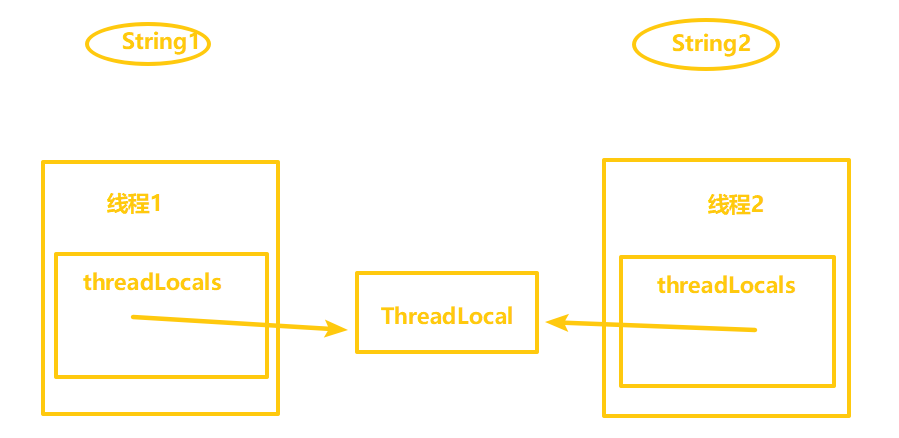java```package test;

static void print(String str) {
//打印当前线程中本地内存中本地变量的值
System.out.println(str + " :" + localVar.get());
//清除本地内存中的本地变量
localVar.remove();
}

public static void main(String[] args) {
@Override
public void run() {
//设置线程1中本地变量的值
localVar.set("localVar1");
//调用打印方法
//打印本地变量
System.out.println("after remove : " + localVar.get());
}
});

@Override
public void run() {
//设置线程1中本地变量的值
localVar.set("localVar2");
//调用打印方法
//打印本地变量
System.out.println("after remove : " + localVar.get());
}
});

t1.start();
t2.start();
}
}``````ThreadLocal.ThreadLocalMap threadLocals=null;### 1、set方法源码

```public void set(T value) {
//(1)获取当前线程（调用者线程）
//(2)以当前线程作为key值，去查找对应的线程变量，找到对应的map
if (map != null)
map.set(this, value);
//(4)如果map为null，说明首次添加，需要首先创建出对应的map
else
createMap(t, value);
}```

```ThreadLocalMap getMap(Thread t) {
}```

```void createMap(Thread t, T firstValue) {
}```

### 2、get方法源码

```public T get() {
//(1)获取当前线程
if (map != null) {
if (e != null) {
@SuppressWarnings("unchecked")
T result = (T)e.value;
return result;
}
}
return setInitialValue();
}

private T setInitialValue() {
//protected T initialValue() {return null;}
T value = initialValue();
//获取当前线程
//以当前线程作为key值，去查找对应的线程变量，找到对应的map
//如果map不为null，就直接添加本地变量，key为当前线程，值为添加的本地变量值
if (map != null)
map.set(this, value);
//如果map为null，说明首次添加，需要首先创建出对应的map
else
createMap(t, value);
return value;
}```

### 3、remove方法的实现

```public void remove() {
if (m != null)
m.remove(this);
}``````package test;

public static void main(String[] args) {
//在main线程中添加main线程的本地变量
//新创建一个子线程
@Override
public void run() {
}
});
//输出main线程中的本地变量值
}
}```

```public class InheritableThreadLocal<T> extends ThreadLocal<T> {

protected T childValue(T parentValue) {
return parentValue;
}

}

void createMap(Thread t, T firstValue) {
}
}```

```private void init(ThreadGroup g, Runnable target, String name,
long stackSize) {
init(g, target, name, stackSize, null, true);
}
private void init(ThreadGroup g, Runnable target, String name,
long stackSize, AccessControlContext acc,
//判断名字的合法性
if (name == null) {
throw new NullPointerException("name cannot be null");
}

this.name = name;
//(1)获取当前线程(父线程)
//安全校验
SecurityManager security = System.getSecurityManager();
if (g == null) { //g:当前线程组
if (security != null) {
}
if (g == null) {
}
}
g.checkAccess();
if (security != null) {
if (isCCLOverridden(getClass())) {
security.checkPermission(SUBCLASS_IMPLEMENTATION_PERMISSION);
}
}

this.group = g; //设置为当前线程组
this.daemon = parent.isDaemon();//守护线程与否(同父线程)
this.priority = parent.getPriority();//优先级同父线程
if (security == null || isCCLOverridden(parent.getClass()))
else
this.inheritedAccessControlContext =
acc != null ? acc : AccessController.getContext();
this.target = target;
setPriority(priority);
this.stackSize = stackSize;

}```

```static ThreadLocalMap createInheritedMap(ThreadLocalMap parentMap) {
}

Entry[] parentTable = parentMap.table;
int len = parentTable.length;
setThreshold(len);
table = new Entry[len];

for (int j = 0; j < len; j++) {
Entry e = parentTable[j];
if (e != null) {
@SuppressWarnings("unchecked")
if (key != null) {
//调用重写的方法
Object value = key.childValue(e.value);
Entry c = new Entry(key, value);
int h = key.threadLocalHashCode & (len - 1);
while (table[h] != null)
h = nextIndex(h, len);
table[h] = c;
size++;
}
}
}
}```

### 1、基础概念

①强引用：Java中默认的引用类型，一个对象如果具有强引用那么只要这种引用还存在就不会被GC。

②软引用：简言之，如果一个对象具有弱引用，在JVM发生OOM之前（即内存充足够使用），是不会GC这个对象的；只有到JVM内存不足的时候才会GC掉这个对象。软引用和一个引用队列联合使用，如果软引用所引用的对象被回收之后，该引用就会加入到与之关联的引用队列中

④虚引用：虚引用是所有引用中最弱的一种引用，其存在就是为了将关联虚引用的对象在被GC掉之后收到一个通知。（不能通过get方法获得其指向的对象）`private Entry[] table;`

```/**
* 是继承自WeakReference的一个类，该类中实际存放的key是
* 由于是弱引用，当get方法返回null的时候意味着坑能引用
*/
static class Entry extends WeakReference<ThreadLocal<?>> {
Object value;

super(k);
value = v;
}
}
//WeakReference构造方法(public class WeakReference<T> extends Reference<T> )
public WeakReference(T referent) {
}

//Reference构造方法
Reference(T referent) {
}

Reference(T referent, ReferenceQueue<? super T> queue) {
this.referent = referent;
this.queue = (queue == null) ? ReferenceQueue.NULL : queue;
}```